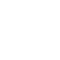# Computer Controlled CMM – Co-ordinate Measuring Machine

The below fig shows the layout of a computer controlled CMM, a flexible measuring centre. The measurements, inspection of parts for dimension form, surface characteristics and position of geometrical elements are done at the same time.

Mechanical systems for the computer controlled CMM can be subdivided into four basic types. The selection of a particular type depends on its application.

The types are,

1. Column type.
2. Bridge type.
3. Cantilever type.
4. Gantry type.

These are shown in fig. The probe used in all these computer controlled CMM may be trigger type or measuring type. It is connected to the spindle in Z direction. The probe establishes the relation between the probing points in the measuring volume and the machine co-ordinate systems. The main features of this system are shown in fig.

#### Trigger type probe system

The main features of these systems are shown in fig. The “buckling mechanism” is a three point bearing, the contacts of which are arranged at 120° around the circumference. These contacts act as electrical micro switches. When being touched in any probing direction, one or more contacts are lifted off and the current is broken, thus it generates a pulse. If the circuit is opened, the current co-ordinate positions are read and stored. After probing, a prestressed spring ensures the perfect zero position of the three point bearing.

The probing force is determined by the prestressed force of the spring. The measuring time is shorter than in static principle, because of the probe system with data acquisition is always dynamic in nature.

#### Measuring type probe system

Measuring type probe mechanism is a small co-ordinate measuring machine in itself. The “buckling mechanism” of this system consists of parallel guide ways as shown in below fig. At the moment of probing, the spring parallelograms are deflected from their initial position.

The measurements can be made easily because the entire system is free from torsion, play and friction. A defined parallel displacement of probes as compared to their original arrangements can be measured.

The displacement of parallelogram is shown in fig. In the static operation, the electronic position regulating mechanism on probing of the specimen moves the slide of the probe axes till the measuring systems of the probes are at their corresponding zero position. After fixing the zero position, the machine coordinates are automatically transferred to the computer.

The mathematical model is used to find the error correction by numerical methods in CMM. A software program gives the measurement of the systematic deviations of the CMM components and string the deviations and on line correction of the measurement valves.

The mathematical model of the mechanical system describes the spatial interaction of the systematic deviations of the CMM components.

If components of CMM are assured as rigid bodies, the position deviations of carriage can be described by the three displacement deviations parallel to the axes (1), (2) and (3) by three rotational deviations about the axes (4), (5) and (6). These are all shown in fig.

The deviation 7 -12 occurs for X – carriage and (13) to (18) for Z – carriage. Thus, 18 path dependent deviations (19), (20) and (21) are to be measured and to be treated in the mathematical model.

The fig shows the effects of systematic deviations of the Y – carriage. The probe stylus in the Y – direction on a line passing through the origin of the coordinate systems (line L1) is not a straight line but a curved one due to errors in the guide. Due to three displacement deviations, the coordinates X, Y, and Z have to be corrected.

By moving on measuring line L2 further, corrections are required due to the offsets X and Z from curve L1 resulting from the pitch angle 5, the roll angle 4 and the yaw angle 6.

In the same way, the deviations of all three carriages and the squareness errors can be taken into account.

The effect of error correction can be tested by means of calibrated step gauges.

The following accuracy/test items are carried out for CMM.

• Measurement accuracy.
• Axial length measuring accuracy.
• Volumetric length measuring accuracy.
• Axial motion accuracy.
• Linear displacement accuracy.
• Straightness.
• Perpendicularity.
• Pitch. Yaw and roll.

The axial length measuring accuracy is tested at the lowest position of the Z-axis on the opposite side of the main axial guide of CMM. The length tested are approximately (1/10), (1/5), (2/5), (3/5) and (4/5) of the measuring range of each axis of CMM. The same test is repeated for five times in each measuring length and results plotted and value of measuring accuracy is derived.